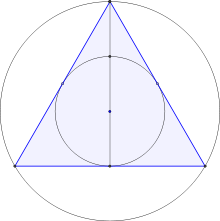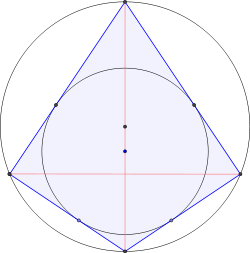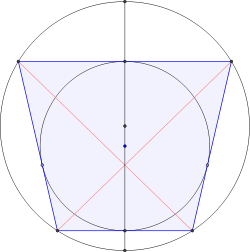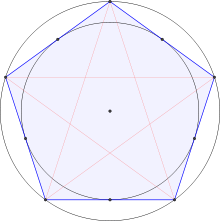# Bicentric polygonIn geometry, a bicentric polygon is a tangential polygon (a polygon all of whose sides are tangent to an inner incircle) which is also cyclic — that is, inscribed in an outer circle that passes through each vertex of the polygon. All triangles and all regular polygons are bicentric. On the other hand, a rectangle with unequal sides is not bicentric, because no circle can be tangent to all four sides.

## Triangles

Every triangle is bicentric. In a triangle, the radii r and R of the incircle and circumcircle respectively are related by the equationwhere x is the distance between the centers of the circles. This is one version of Euler's triangle formula.

Not all quadrilaterals are bicentric (having both an incircle and a circumcircle). Given two circles (one within the other) with radii R and r where, there exists a convex quadrilateral inscribed in one of them and tangent to the other if and only if their radii satisfywhere x is the distance between their centers. This condition (and analogous conditions for higher order polygons) is known as Fuss' theorem.

## Polygons with n > 4

A complicated general formula is known for any number n of sides for the relation among the circumradius R, the inradius r, and the distance x between the circumcenter and the incenter. Some of these for specific n are:whereand## Regular polygons

Every regular polygon is bicentric. In a regular polygon, the incircle and the circumcircle are concentricthat is, they share a common center, which is also the center of the regular polygon, so the distance between the incenter and circumcenter is always zero. The radius of the inscribed circle is the apothem (the shortest distance from the center to the boundary of the regular polygon).

For any regular polygon, the relations between the common edge length a, the radius r of the incircle, and the radius R of the circumcircle are:For some regular polygons which can be constructed with compass and ruler, we have the following algebraic formulas for these relations:3456810Thus we have the following decimal approximations:## Poncelet's porism

If two circles are the inscribed and circumscribed circles of a single bicentric n-gon, then the same two circles are the inscribed and circumscribed circles of infinitely many bicentric n-gons. More precisely, every tangent line to the inner of the two circles can be extended to a bicentric n-gon by placing vertices on the line at the points where it crosses the outer circle, continuing from each vertex along another tangent line, and continuing in the same way until the resulting polygonal chain closes up to an n-gon. The fact that it will always do so is implied by Poncelet's closure theorem, which more generally applies for inscribed and circumscribed conics.

## References

1. Gorini, Catherine A. (2009), The Facts on File Geometry Handbook, Infobase Publishing, p. 17, ISBN 9780816073894.
2. Reiman, István (2005), International Mathematical Olympiad: 1976-1990, Anthem Press, pp. 170–171, ISBN 9781843312000.
3. Davison, Charles (1915), Subjects for mathematical essays, Macmillan and co., limited, p. 98.
4. Dörrie, Heinrich (1965), 100 Great Problems of Elementary Mathematics: Their History and Solution, Courier Dover Publications, p. 192, ISBN 9780486613482.
5. Weisstein, Eric W. "Poncelet's Porism." From MathWorld--A Wolfram Web Resource. http://mathworld.wolfram.com/PonceletsPorism.html
6. Flatto, Leopold (2009), Poncelet's Theorem, American Mathematical Society, ISBN 9780821886267.